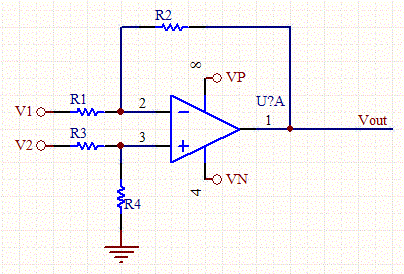# Op-Amp Voltage and Gain Calculator

This calculator determines the output voltage and gain for an op-amp, given it's resistor values, and DC input values. The output voltage is independent of whether the op-amp is used in the inverting or non-inverting configuration. It's easy to figure out the characteristics for an AC signal given the output bias point and gain.

For a traditional, non-inverting op-amp just set V1 to 0V and use V2 as the input, and for inverting set V2 to 0v and use V1 as the input. If R3 isn't used, the use a very large value, for infinite resistance.Op-Amp Circuit Schematic

 Input Parameters R1 (KOhms) R2 (KOhms) R3 (KOhms) R4 (KOhms) V1 (V) V2 (V) Vp (V) Vn (V) Outputs Vout (V) Output is clipped. Gain Inverting (Vout/V1) Gain non-inverting (Vout/V2)